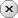### 用户注册### 用户登录### 发表随想lb0123    -  云代码空间

—— 自己 不努力每人会帮你

# python学习笔记

2020-01-28|52阅||

## 摘要:变量与函数

```#数学函数

#abs  求绝对值
a=-2
print('a的绝对值为:',abs(a))

#max  求最大值
print ('最大值为：',max(1,2,3,4,5)) #结果为：5

#min  求最小数
print ('最小值为：',min(1,2,3,4,5)) #结果为：1

#pow  求次方运算
print('求次方为：',pow(2,4))   #结果为：16

#round 四舍五入运算
print('四舍五入的结果为：',round(3.1415926,4)) #其中保留几位小数的4可以省略，若省略则默认保留整数
#结果为：3.1416

#数学模块
import math #导入数学模块   使用数学模块必须先导入数学模块

#math.ceil() 向上取整模块
print('向上取整的结果为：',math.ceil(1.84))  #结果为：2

#math.floor() 向下取整模块
print('向下取整的结果为：',math.floor(1.84))  #结果为：1

#math.modf 得到浮点数的整数和小数部分
print('得到的浮点数整数和小数部分非别为：',math.modf(3.1415926)) #结果为：(0.14159260000000007, 3.0)  注意存在误差

#math.sqrt 开方运算
print('得到的开方结果为：',math.sqrt(4))  #结果为：2.0

#随机数模块
import random #导入随机数模块，随机数使用之前都需要导入随机数模块

#random.choice 从指定数字中输出随机数
print('得到的随机数为：',random.choice([12,3,4,5,6,])) #从[]所包含的所有数中随机打印出一个数字

#random.randrange([start],[stop],[step])   从一个范围内取出一个随机数其中随机数开始为start结束于stop各数间隔为step
print('得到的随机数为：',random.randrange(1,10,1)) #在1——10中随机输出一个数其中取数间隔为1，其中不包括10，但是包括1，但是间隔不能为小数

#random.random 在[0,1)之间随机产生一个浮点数
print('在0-1之间随机产生一个浮点数：',random.random())  #产生的随机数在0-1之间，且包括0不包括1

#random.unifor() 在范围内随机产生一个浮点数
print('3-8之间的随机数为：',random.uniform(3,8))  #产生的浮点数在3-8之间

#random.shuffle() 将序列的元素随机排列
arr = [0,1,2,3,4,5,6,7,8,9]
random.shuffle(arr)
print('将数列随机排序为：',arr) #将arr中的序列随机排列

#random.randint(start,stop) 在start-stop之间随机产生一个整数，且包含start和stop
print('1-5之间产生的随机数为：',random.randint(1,5))  #在1-5之间随机产生一个整数

#数据类型转换
print('1.3转换成int为：',int(1.3)) #将1.3转换成int型的数 结果为1

print('将1转换成浮点数为：',float(1)) #将1转换成浮点数

#算术运算：+ -  * / % ** //
num1 = 2
num2 = 5
print('num1+num2=',num1+num2)
print('num1-num2=',num1-num2)
print('num1*num2=',num1*num2)
print('num1/num2=',num1/num2)
print('num1%um2=',num1%num2)
print('num1**num2=',num1**num2)
print('num1//num2=',num1//num2)```

概述
1.程序可操作存储区的内容
2.程序运行期间能改变的数据
3.每个人变量都有不同的类型
作用：将不同类型的数据储存到内存中去
定义变量：
变量名 = 数据值
注意：变量在使用前需要先定义，否则会出错。
删除变量：
del 变量名
注意：删除后的变量无法引用
常量：程序运行中不能改变的数据.
1、Number数字
分类：
1.整数
python可以处理任意大小的整数，还包含复数
定义num1 = 10
num2 = num3 = num4 = 10

2.浮点数
浮点数有整数部分和小数部分组成
但在计算时可能有四舍五入的误差产生
3.复数
复数组成包括实数部分和虚数部分
4.数学函数
abs 求绝对值   例如：abs(-2) 求出-2的绝对值
max 求最大值   例如：max(1,2,3,4,5,6) 求出1,2,3,4,5,6中的最大值
min 求最小值   例如：min(1,2,3,4,5,6) 求出1,2,3,4,5,6中的最小值
pow 求次方     例如：pow(2,4) 表示2的4次方
round 四舍五入 例如：round(3.1415926,4) 表示将3.1415926进行四舍五入，并保留四位有效数字，其中 4可以省略
若省略则表示进行四舍五入到整数，计算结果为 3

5.数学模块
import math 导入数学模块   使用数学模块必须先导入数学模块
math.ceil(18.1)   向上取整  结果：19
math.floor(18.1)  向下取整  结果：18
math.modf(18.1)   得到浮点数的小数部分和整数部分  结果：(0.10000000000000142, 18.0)
math.sqrt(5)       开方被开方数不能为负数   结果：2.23606797749979

6.随机数
import random 导入随机数模块  使用随机数函数必须先导入随机数模块
random.choice([12,3,4,5,6,]) 从指定数字中随机输出一个数
randrange([start],[stop],[step])  在一定范围内随机数出一个数，这个随机数开始于start，结束于stop但不包括stop，每个数字间隔为step若无start默认从0开始若无step指定的基数为1
random.random 在[0，1）之间随机生成一个数
random.uniform(a,b)  在a，b之间随机产一个浮点数
random.shffile(arr)  arr = [ , , , , ] 对arr这个数组（序列）随机排序
random.randint(start,stop) 在start-stop之间随机产生一个整数，且包含start和stop
7.三角函数
asin() 反正弦弧度值
atan() 反正切弧度值
acos() 反余弦弧度值
cos()  弧度的余弦值
sin()  弧度的正弦值
tan()  弧度的正切值
cot()  弧度的余切值
8.数据类型转换
int(x) 将x转换成一个整数
float(x) 将一个数转换成一个浮点数

发表评论

• 昵称： lb0123
• 等级： 中级程序员
• 积分： 257
• 代码： 7 个
• 文章： 8 篇
• 随想： 0 条
• 访问： 0 次
• 关注